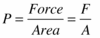Physics2 7K Forces Flashcards Preview

Year 7 Science > Physics2 7K Forces > Flashcards

Flashcards in Physics2 7K Forces Deck (20):
1

What things could a force do to an object?2

Do forces have just size, just direction, or size and direction?

Forces have size and direction.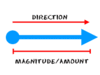3

On a diagram, what can we use to show a force?

On a diagram you can use an arrow to show a force.The length of the arrow shows the size of the force, and the direction the arrow is pointing shows the direction the force will act.4

What is meant by a contact force?

A contact force is one in which objects are touching. For example, kicking a ball, or friction.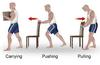5

What is meant by a non-contact force?

A non-contact force is one where the objects are not touching. For example, magnetism and gravity.6

What is friction?

Friction is a force that slows things down. It always opposes movement.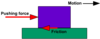7

What can you use to reduce friction?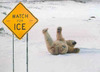To reduce friction, you can use lubrication. for example, oil on a bike chain.8

What is meant by balanced forces?

Balanced forces are forces which cancel each other out, so there is no change in movement.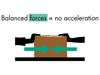9

What is the name of the force labelled X?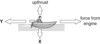10

What is the name of the force labelled Y?11

If the force from the engine is bigger than force Y, what happens to the boat?12

What can you say about the size of force X compared to the upthrust?13

What is meant by pressure?

Pressure is a measurement of how spread out a force is.14

Explain, using ideas about pressure, why wading birds have webbed feet.15

16

Calculate the pressure when a boy of weight of 400 N sits on a chair with an area of 0.25 m2.17

What causes air resistance?

Air resistance is caused by friction between the air particles and the moving object.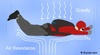18

What happens to air resistance if you are travelling faster?

If you are travelling faster, air resistance increases.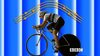19

Why do mountain bikes have wide tyres?20

What is the formula to calculate pressure?